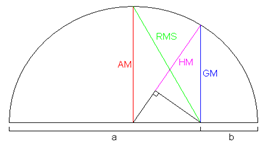×#### Thank you for registering.

One of our academic counsellors will contact you within 1 working day.

Click to Chat

1800-1023-196

+91-120-4616500

CART 0

• 0

MY CART (5)

Use Coupon: CART20 and get 20% off on all online Study Material

ITEM
DETAILS
MRP
DISCOUNT
FINAL PRICE
Total Price: Rs.

There are no items in this cart.
Continue Shopping```Harmonic ProgressionHarmonic progression is an important topic of sequence and series which is closely related to the arithmetic progression. We shall discuss arithmetic and geometric progressions in brief as they have been discussed in detail in the earlier sections.

Arithmetic Progression: A progression in which there is a fixed difference between every pair of two terms i.e. the progression of the form a, a+d, a+2d, …..  is known as an arithmetic progression.

Geometric Progression: The sequence or progression of the form a, ar, ar2, …. is said to be a geometric progression.

Harmonic Progression

The simplest way to define a harmonic progression is that if the inverse of a sequence follows the rule of an arithmetic progression then it is said to be in harmonic progression. This simply means that if a, a+d, a+2d, …..   is an A.P. then 1/a, 1/(a+d), 1/(a+2d), …… is an H.P.

For example, the series 1 +1/4 +1/7 +1/10 +..... is an example of harmonic progression, since the series obtained by taking reciprocals of its corresponding terms i.e. 1 +4 +7 +10 +... is an A.P.

Harmonic Mean

Let a, b and c form an H.P. Then as discussed above, 1/a, 1/b and 1/c form an A.P. Then 2/b = 1/a + 1/c. Hence, b = 2ac/ (a+c). This ‘b’ is called as the harmonic mean of a and c.

Illustration: We have a H.P. whose 7th term is 1/10 and 12th term is 1/25. Find the 20th term and hence the nth term.

Solution: We know that the general form of H.P. is

1/a + 1/(a + d) + 1(a + 2d) + ...
The 7th term = 1/(a + 6d) = 1/10 =>  a +6 d = 10
The 12th term = 1/(a + 11d) = 1/25  => a +11 d = 25
Solving these two equations, a = -8, d = 3
Hence 20th term = 1/(a+19d) = 1/[-8 + 9(3)] = 1/49
and nth term = 1/[a +(n -1)d] = 1/[-8 +(n -1) 3] = 1/[3n - 11]

Illustration: If a, b, c are in H.P., show that a/b+c, b/c+a, c/a+b are also in H.P.

Solution: It is given in the question that a, b and c are in H.P.

By definition it follows that 1/a, 1/b, 1/c are in A.P

⇒ (a+b+c)/a, (a+b+c)/b, (a+b+c)/c are in A.P.

⇒ 1+ (b+c)/a, 1 + (c+a)/b, 1 + (a+b)/c are in A.P

⇒ (b+c)/a, (c+a)/b, (a+b)/c are in A.P.

⇒ a/b+c, b/c+a, c/a+b are in H.P.

Illustration: If a2, b2 and c2 are in A.P., then prove that b+c, c+a, a+b are in H.P.

Solution: It is given that a2, b2 and c2 are in A.P.

Hence, adding (ab + bc + ca) to each term

So, a2 + ab + bc + ca, b2 + ab + bc + ca and c2 + ab + bc + ca will also be in A.P

Hence, a(a+b) + c(a+b), b(a+b) + c(a+b), c(c+b) + a(b+c) are in A.P

i.e. (a+b) (a+c), (a+b) (c+b), (a+c) (c+b) are in A.P

dividing each term by (a+b) (c+b) (a+c) we get,

1/b+c, 1/c+a, 1/a+b are in A.P

Hence, (b+c), (c+a), (a+b) are in H.P.

Some Important Points

As the nth term of an A.P is given by an = a + (n-1)d,

So the nth term of an H.P is given by 1/ [a + (n -1) d].

Let a and b be two positive real numbers, then

A.M x H.M = G.M2

The relation between the three types of means is

A.M > G.M > H.M.

The figure given below further illustrates this relationRelation Between A.M, G.M and H.M

If we need to find three numbers in a H.P. then they should be assumed as 1/a–d, 1/a, 1/a+d

Four convenient numbers in H.P. are

1/a–3d, 1/a–d, 1/a+d, 1/a+3d

Five convenient numbers in H.P. are

1/a–2d, 1/a–d, 1/a, 1/a+d, 1/a+2d

Majority of the questions of H.P. are solved by first converting them into
A.P.

askIITians offers extensive study material which covers all the important topics of IIT JEE Mathematics. Units like harmonic progression and arithmetic progression have been covered in detail along with several solved examples. The JEE aspirants must have a strong grip on the topics in order to remain competitive in the JEE.

Related Resources

Look into the Past Year Papers with Solutions to get a hint of the kinds of questions asked in the exam.

You can get the knowledge of Useful Books of Mathematics here.

You may also like to refer Arithmetic Mean and Geometric Mean.

To read more, Buy study materials of Sequences and Series comprising study notes, revision notes, video lectures, previous year solved questions etc. Also browse for more study materials on Mathematics here.
```### Course Features

• 731 Video Lectures
• Revision Notes
• Previous Year Papers
• Mind Map
• Study Planner
• NCERT Solutions
• Discussion Forum
• Test paper with Video Solution